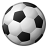# QlikView App Dev

Discussion Board for collaboration related to QlikView App Development.

Announcements
An issue has been identified on Qlik Cloud hub, please visit our Status Update Page for details: GET THE LATEST
cancel
Showing results for
Did you mean:Specialist

## Pick and Match

Hi,

I have a requirement which is as follows:

I have Warehouse, ID, Units

Where Warehouse comes from Table1 and ID and Units were from Table2.

When i select Warehouse from Table1, Count(Distinct(ID) from Table 2and Sum(Units) from Table2 should be displaye d in table.

=Pick(Match([W1], 'ABC'), Sum(TOTAL{<W2= {'ABC'}>} [Units]))

=Pick(Match([W1], 'ABC'), Count(Distinct{<W2= {'ABC'}>} ID))

The above coed is not giving me right answer...

Can anyone look into this.

14 RepliesMVP

thanks

regards

MarcoMVP

What do you mean with 'not giving right answer'?

If you don't tell us by, it's just guessing around.

And what is field W1, maybe the Warehouse field?

If you select more then a single W1 value or you don't have any selection on this field, Match([W1],'ABC') will obviously not work even when 'ABC' is part of the possible values.

It's most of the time easier to help you if you could post a small sample QVW, or a sample INLINE data set and your expected result.Specialist III

=Pick(Match([W1], 'ABC')+1, Null(),Sum(TOTAL{<W2= {'ABC'}>} [Units]))

=Pick(Match([W1], 'ABC')+1, Null(),Count(Distinct{<W2= {'ABC'}>} ID))Specialist
Author

Hi,

Here is the sample data.Specialist
Author

Hi Sir,

I tried it.

is =Pick(Match([W1], 'ABC')+1, Null(),Sum(TOTAL{<W2= {'ABC'}>} [Units]))

same as

=Pick(Match([W1], 'a'), Sum(TOTAL{<W2= {'a'}>} [units])) ???

Can u pls lemme knw about the logic??Luminary Alumni

Hi,

Instead of joining you can use Concatenate() like below

Table:

W1 AS Warehouse,

units,

'Table1' AS Source

FROM

Book1.xlsx

(ooxml, embedded labels, table is Sheet1);

Concatenate(Table)

W2 AS Warehouse,

units,

'Table2' AS Source

FROM

Book2.xlsx

(ooxml, embedded labels, table is Sheet1);

Now W1 and W2 are in single field and values are summed up when selected in this field.

To get Count and Sum of values use below expressions

Count({<Source={'Table2'}>}Distinct ID)

Sum({<Source={'Table2'}>}Distinct Units)

Regards,

Jagan.Specialist III

Match([W1], 'ABC') returns zero if W1 is not equal to ABC.

pick(n, expr1[ , expr2,...exprN])

Returns the n:th expression in the list. n is an integer between 1 and N.

So in order to make the first number 1, I am adding 1 to the result of Match().Specialist
Author

Hi Sir,

Here is the application...

Sum is working but not Count....Specialist
Author

using the same pick and match functions, can i please know how can i diaply the values of LBCD.

=Pick(Match([W1], 'OB1')+1, Null(),Count(Distinct{<[W2]= {'OB1'}>} LBCD))Community Browser